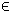## 23GOAL Problem

The constrained goal attainment (goal) problem is defined as

 min x
max lam: r(x) − w * lamg
subject to xL x xU
bL Ax bU
cL c(x) cU
(25)
where x,xL,xURn, r(x)RN, c(x),cL,cURm1, bL,bURm2, ARm2 × n, gRm, and wRm.

The goal solution can be obtained by the use of any suitable nonlinear TOMLAB solver.

The following files define a problem in TOMLAB.

File: tomlab/quickguide/goalsQG_r.m, goalsQG_J.m, goalsQG_c, goalsQG_dc
```  r:   Residual vector
J:   Jacobian matrix
c:   Nonlinear constraint vector
```
The following file illustrates how to solve a goal attainment problem in TOMLAB. Also view the m-files specified above for more information.

File: tomlab/quickguide/goalsQG.m

Open the file for viewing, and execute goalsQG in Matlab.
```% goalsQG is a small example problem for defining and solving
% multi criteria optimization problems using the TOMLAB format.

Name='EASY-TP355';
% Constrained least squares problem, four quadratic terms and local solutions
% Hock W., Schittkowski K. (1981):
x_0 = zeros(4,1);    % Lower bounds for x.
x_L = zeros(4,1);    % Upper bounds for x.
x_U = 1e5*ones(4,1); % Starting point.
x_min = [];          % For plotting.
x_max = [];          % For plotting.
A   = [1 0 0 0;0 1 0 0];  % Linear constraints.
b_L = [0.1;0.1];          % Lower bounds.
b_U = [0.1;0.1];          % Upper bounds.
c_L = 0;                  % Lower bounds.
c_U = 0;                  % Upper bounds.
y   = zeros(2,1);         % Residuals

Prob = clsAssign('goalsQG_r', 'goalsQG_J', [], x_L, x_U, Name, x_0,...
y, [], [], [], [], [],...
A, b_L, b_U, 'goalsQG_c', 'goalsQG_dc', [], c_L, c_U,...
x_min, x_max);

PriLev = 2;
Result = tomRun('goalSolve', Prob, PriLev);```